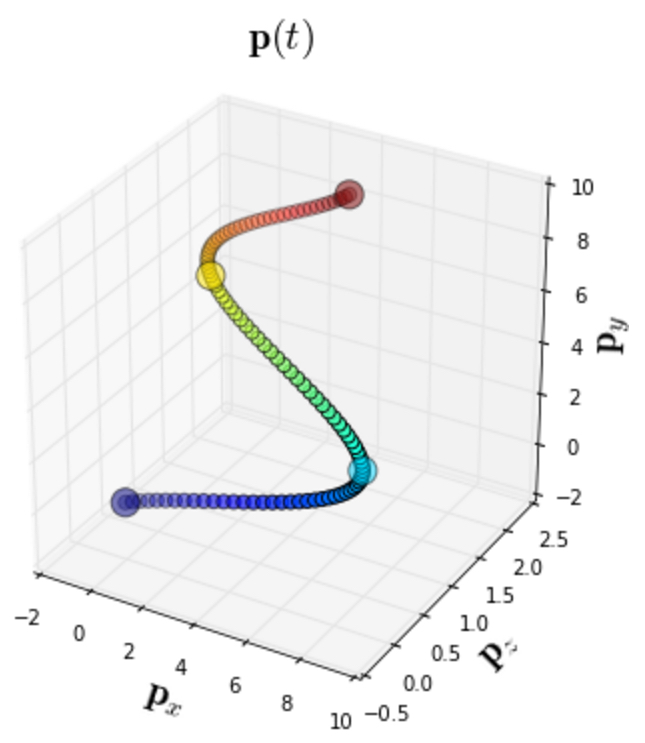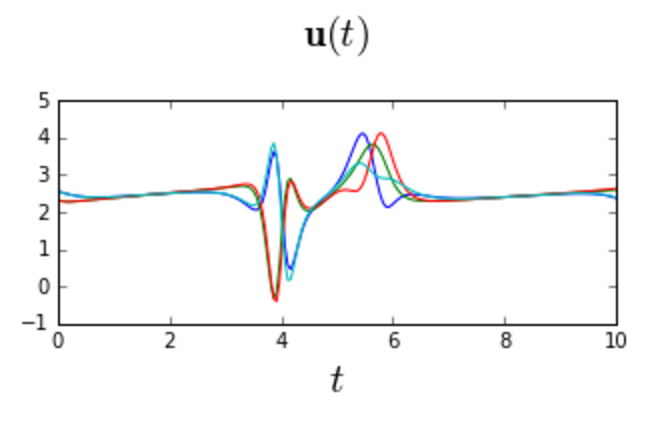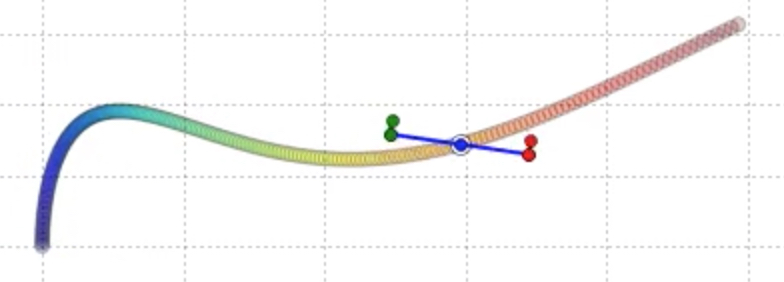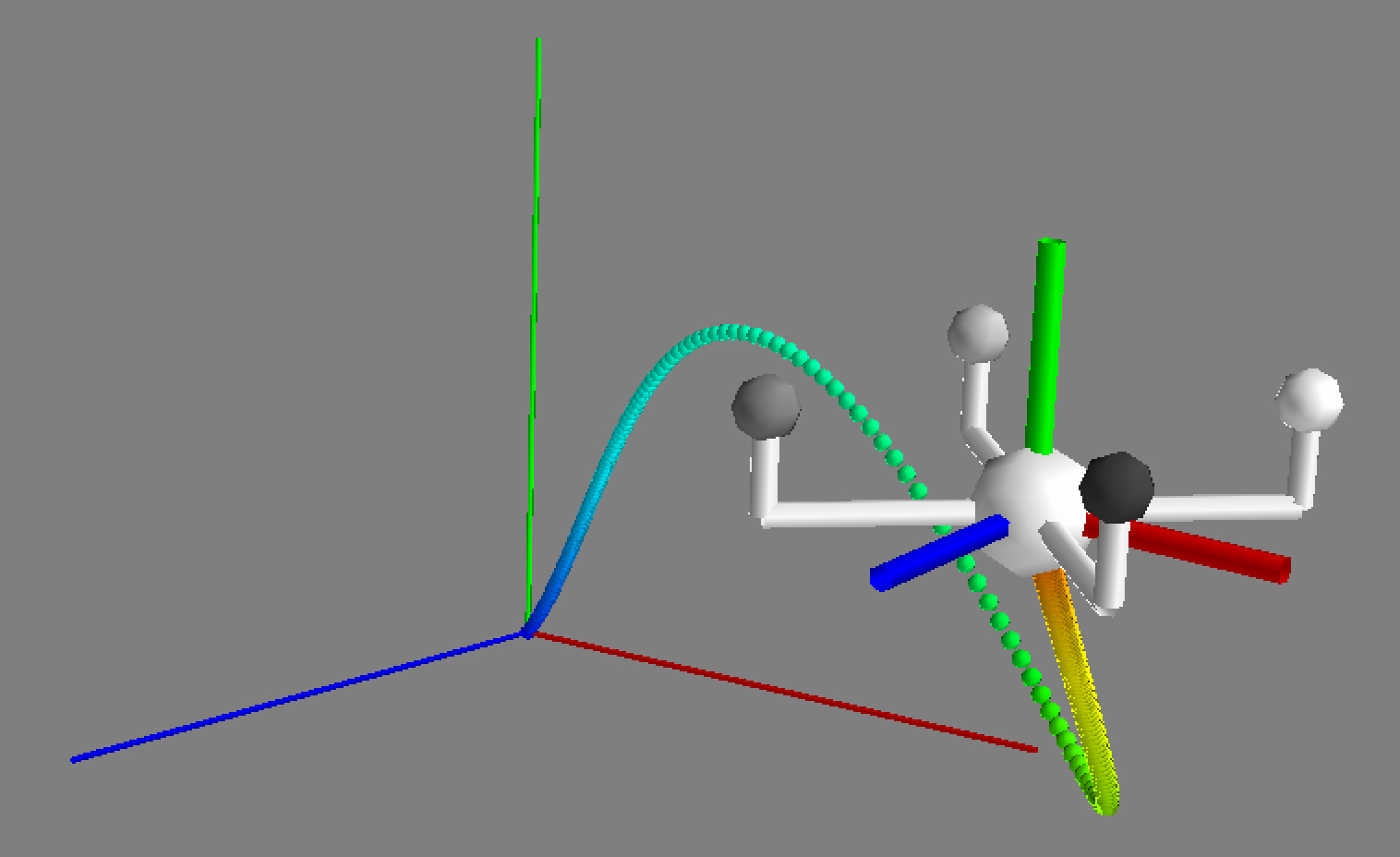# Welcome to Flashlight¶

Flashlight is a lightweight Python library for analyzing and solving quadrotor control problems. Flashlight enables you to easily solve for minimum snap trajectories that go through a sequence of waypoints, compute the required control forces along trajectories, execute the trajectories in a physics simulator, and visualize the simulation results. Flashlight also makes it easy to simulate external disturbances, and to recover from those disturbances using time-varying LQR feedback control. Flashlight includes physical models for 2D quadrotors, 3D quadrotors, and 3D quadrotor cameras.The following code snippet shows how easy it is to start analyzing quadrotor trajectories using Flashlight. In this code snippet, we generate the control forces required for a 2D quadrotor to follow a simple trajectory, and simulate the results:

from pylab import *; import scipy.integrate

import flashlight.interpolate_utils as interpolate_utils

# Define a simple position trajectory in 2D.
num_samples = 200
t_begin     = 0
t_end       = pi
dt          = (t_end - t_begin) / (num_samples - 1)

t = linspace(t_begin, t_end, num_samples)
p = c_[ sin(2*t) + t, t**2 ]

# Compute the corresponding state space trajectory and control trajectories for a 2D quadrotor.
q_qdot_qdotdot = quadrotor_2d.compute_state_space_trajectory_and_derivatives(p, dt)

# Define a function that interpolates the control trajectory in between time samples.
u_interp_func = interpolate_utils.interp1d_vector_wrt_scalar(t, u, kind="cubic")

# Define a simulation loop.
def compute_x_dot(x_t, t):

# Get the current control vector.
u_t = u_interp_func(clip(t, t_begin, t_end))

# Compute the state derivative from the current state and current control vectors.
x_dot_t = quadrotor_2d.compute_x_dot(x_t, u_t).A1

return x_dot_t

# Simulate.
x_nominal, _, _, _ = quadrotor_2d.pack_state_space_trajectory_and_derivatives(q_qdot_qdotdot)
x_0                = x_nominal
x_sim              = scipy.integrate.odeint(compute_x_dot, x_0, t)

# Plot the results.
quadrotor_2d.draw(t, x_sim, t_nominal=t, x_nominal=x_nominal, inline=True)


This code example produces the following animation, which shows our 2D quadrotor correctly following the intended trajectory:

Flashlight is designed and implemented by Mike Roberts.

# Installing Flashlight¶

The steps for installing Flashlight are as follows.

1. Install all of Flashlight’s dependencies. The core functionality in Flashlight depends on the following Python libraries:
Many of the example notebooks, and some of the debug rendering functions in Flashlight, depend on the following Python libraries:

Each of these dependencies comes pre-installed with Enthought Canopy, or can be installed very easily using the using the Enthought Canopy package manager, or pip. The only dependency that is slightly more involved to install is Slycot, but this is still easy to install, by downloading the source from GitHub and running sudo python setup.py install from the top-level GitHub folder.

1. Download the Flashlight source code from our GitHub repository.

2. In any folder you’d like to use Flashlight, copy our path/to/flashlight/code/utils/path_utils.py file into that folder.

3. Include the following code snippet in your Python code before importing Flashlight:

import path_utils

4. Verify that you can import flashlight from your Python code. This import statement should print Initializing flashlight v0.0.1 to the console.

After completing these steps, you’re ready to start using Flashlight.

# Reproducible Research¶

As part of the Flashlight source code, we include notebooks that reproduce the experimental results from our research papers.

Niels Joubert, Mike Roberts, Anh Truong, Floraine Berthouzoz, Pat Hanrahan
ACM Transactions on Graphics 34(6) (SIGGRAPH Asia 2015)

# Projects using Flashlight¶

Flashlight is being used in the following projects:

Mike Roberts, Pat Hanrahan
ACM Transactions on Graphics 35(4) (SIGGRAPH 2016)
Niels Joubert, Mike Roberts, Anh Truong, Floraine Berthouzoz, Pat Hanrahan
ACM Transactions on Graphics 34(6) (SIGGRAPH Asia 2015)

# Citing Flashlight¶

If you use Flashlight for published work, we encourage you to cite it as follows:

@misc{flashlight:2016,
author       = {Mike Roberts},
title        = {Flashlight: A Python Library for Analyzing and Solving Quadrotor Control Problems},
year         = {2016},
howpublished = {\url{http://mikeroberts3000.github.io/flashlight}}
}


Additionally, if you use any of the functionality in curve_utils, quadrotor_3d, quadrotor_camera_3d, or spline_utils for published work, we encourage you to cite the following paper:

@article{joubert:2015,
author  = {Niels Joubert AND Mike Roberts AND Anh Truong AND Floraine Berthouzoz AND Pat Hanrahan},
title   = {An Interactive Tool for Designing Quadrotor Camera Shots},
journal = {ACM Transactions on Graphics (SIGGRAPH Asia 2015)},
volume  = {34},
number  = {6},
year    = {2015}
}